# 2nd PUC Basic Maths Question Bank Chapter 11 Linear Programming Problems

Students can Download Basic Maths Question Bank Chapter 11 Linear Programming Problems Questions and Answers, Notes Pdf, 2nd PUC Basic Maths Question Bank with Answers helps you to revise the complete Karnataka State Board Syllabus and to clear all their doubts, score well in final exams.

## Karnataka 2nd PUC Basic Maths Question Bank Chapter 11 Linear Programming Problems

### 2nd PUC Basic Maths Linear Programming Problems Four Marks Questions and Answers

Question 1.
A person wants to decide the constituents of a diet which will fulfill his daily requirements of proteins, fats & carbohydrates at a minimum cost. The combination is made among two food products A & B whose contents are indicated below.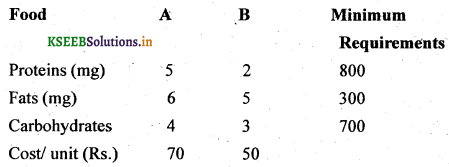Formulate the LPP
Let the units of Food A be x & Food B be y.
Food A requires 5 mg proteins & B required 2 mg of proteins & minm requirements is 800 mg
∴ 5x + 2y ≥ 800
Food A requires 6 mg and B requires 5 mg of fats minimum requirements of fats is 300 ∴ 6.x + 5y ≥ 300
Food A requires 4 mg and B requires 3 mg of carbohydrates, minm requirement of carbohydrates is 700 mg
4x + 3y ≥ 700
The objective function is
Minimise = Z – 70x + 50y
Subject to the constraints
5 x + 2y ≥ 800
6x + 5 y ≥ 300
4x + 3y ≥700
x ≥ 0, y ≥ 0

Question 2.
A Businessmen buys & sells chairs and tables. He has Rs. 3000/- to invest, A chair cost him Rs. 50/- & a table cost Rs. 90/- He has space which can accomodate at most 48 pieces if he sells each chair for Rs. 200 & each table for Rs. 400/- Find the number of chairs and tables he has to buy to obtain maximum profit.
Let the number of chairs be x and the tables by y
cost of one chair is Rs 50/- and one table is Rs. 90/-.
He has Rs. 3000/- to invest.
He can invest less than or equal to 3000 Rs.
∴ 50 x + 90 y < 3000
He has space which can accommodate not more than 48 pieces.
∴ x +y < 48
His aim is to maximise profit and selling of each is Rs. 200 & table is Rs 400
∴ objective function is
Maximise z = 200 x + 400 y
Subject to the constraints
50 x + 90y ≤ 3000
x+y ≤ 48
x ≥ 0, y ≥ 0Question 3.
A manufacturer produces 2 products P and Q. Each P requires 4 hours on machine M & 2 hrs on machine M1. Each Q requires 2 hours an machine M1 and 5 hrs on machine M2 the available total time on M1 is 2 hrs and on M2 is 24 hours profit per unit of P is Rs. 6 and that of Q is Rs. 8 what Quantities of each should be produced and sold to maximize profits formulate the L P P.
Let the number of units of product P be x and number of units of product Q be y P requires 4 hrs on machine M1 2 hrs on M2 total available time on machine is 120 and machine M2 is 24, Q quires 2 hrs on M1 and 5 hrs on M2
∴ 4x + 2y ≤ 120 and 2x + 5y ≤ 24
∴ profit on P is 6 Rs. and Q is 8 Rs.
maximize Z = 6x + 8y
Since negative units cannot be produced, x > 0, y > 0
Max Z = 6x + 8y Subject to the constraints
4x + 2y ≤ 120
2x + 5y ≤ 24
x ≥ 0 , y ≥ 0

Question 4.
Smaran being a manufacturer produces nuts & bolts for industrial machinery. It takes 1 hr of work on machine A and 3 hrs on machine B to produce a package of nuts while it takes 3 hrs on machine A & 1 hr on machine B to produce a package on bolts. He earns a profit of ₹ 2. 50 per package on nuts and ₹1 per package on bolts form a linear programming problem to mizimise his profit, if he operates each machine for at most 12 hrs a day.
Let ‘x’ be the units of nuts produced, & y be the units of bolts produced.
The formulated LPP is given by.
Max. ≥ = 2.5 x + y
Subject to the constraints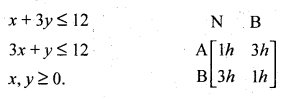Question 5.
Vishal consumes two types of Food, A & B. Every day to obtain minimum 8 units of protein, 12 units carbohydrates and 9 units of fat which is his daily requirements, 1kg of food A contains 2,6,1 units of protein, carbohydrates & fats respectively 1kg of food B contains 1,1, & 3 units of P, C & F respectively. Foods cost’ 8 per kg and food B cost’ 5 per kg. Form an L P P to find how many kg of each food should he buy daily to minimize his cost of food & still meet minimal nutritional requirements.
Let V be the no of units of food A and y be the no of units of food B.
The formulated LPP is given by
Mix z = 8x + 5y
Subject to the constrains.Question 6.
Archana, a dietician wishes to mix two types of foods F1 & F2 in such a way that the vitamin contents of the mixture contains at least 6 units of vitamin A and 8 units of vitamin B. Food F1 contains 2 units 1kg of vitamin and 3 units 1kg of vitamin B while food F2 contains 3 units 1kg of vitamin A and 4 units 1 kg of vitamin B. Food F1 costs X 50 per kg and food F2 costs ₹ 75 per kg formulate the problem as LPP To minimize the cost of the mixture.
Let V be the no of units of F, and ‘y’ be the no of units of F2.
The formulated LPP is given by
Mix z = 50 x + 75 y
Subject to the constraintsQuestion 7.
A furniture maker jatin has 6 units of wood and 28 hrs of free time in which he will make decorative screens two models have sold well in the past so he will restrict himself to those two he estimates that model 1 requires 2 units of wood & 7 hours of time model Q requires 1 unit of wood & 8 hrs of time the prices of the models are ₹ 120 & ₹ 80 respectively. Formulate LPP to determine how many screens of each model should the furniture maker assemble if he wishes to maximize his sales revenue.
Let V be the no of units of Model 1 and y be the no of units of Model 2 the formulated LPP is given by Max Z = 120x + 80y Subject to the constraintsQuestion 8.
A firm owned by sheshnag manufactures two types of products A & B and sell them at a profit of ₹2 on type A and ₹3 on types B. Each product is processed on machines and M2 type A requires one minute of processing time on M1 and two minutes on M2 type B requires one minute of time an M1 & one minute on M2 the machine M1 is available for not more than 6 hours 40 minutes whole M2 is available for 10 hours during any working day. Formulate the LPP in order to find how many products of each type should the firm producer each day so that profit is maximum.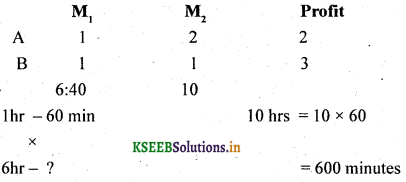= 360 min + 40 min
= 400 minutes
maximize z = 2x + 3y
x + y ≤ 400
2x + y ≤ 600
x ≥ 0,y ≥ 0

Question 9.
Producer Rahul has 50 & 85 units of labour & capital respectively which he can use to produce two types of goods A & B. To produce one units of A, 1 units of labour and 2 units of capital are required. Similarly 3 units of labour and 2 units of capital is required to produce 1 unit of B if A & B are priced at ₹100 & ₹150 per unit respectively, how should the producer use his resources to maximize the total reveue. Formulate the LPP to maximize his total revenue?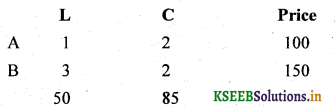Maximize z = 100x + 150y
Subject to constraints
x + 3y ≤ 50
2x + 2y ≤ 85
x,y ≥ 0
Maximize z = 100x + 150y
Subject to constraints
x + 3y ≤ 50
2x + 2y ≤ 85
x,y ≥ 0Question 10.
Nikhil pesticide company must produce 200 kg mixture consisting of chemicals A & B daily A cost ₹ 3 per kg and B cost ₹ 8 per kg. Maximum 80 kg of chemical A & at least 60 kg of chemical B should be used, Formulate LPP model to minimize the cost.
Let the chemical ‘A’ be ‘x’
Let the chemical ‘B’ be ‘y’
Minimize z = 3x+ 8y
Subject to constraints;
x + y ≤ 200
x ≤ 80
y ≥ 60
x,y ≥ 0

### 2nd PUC Basic Maths Linear Programming Problems Five Marks Questions and Answers

(II) Solve the following LPP using graphical method.

Question 1.
Maximise z = 60>x + 15y Subject to constraints
x+y ≤ 50
3x+y ≤ 90
x,y ≥ 0
Let us consider the in equalities as equalities i, e
x+y = 50
Put x = 0, then y = 50, (0, 50)
Put y = 0 then x = 50 (50, 0)

3x + y = 50
Put x = 0, y = 90, (0, 90)
Puty = 0 ,x = 30 (30,0)
Pot the points (i) (0, 50) and (50,0) (ii) (0,90) & (30,0) on the graph shown in the graph.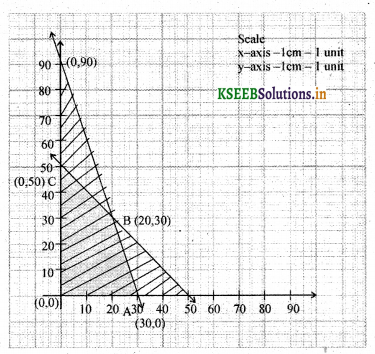The region O A B C is called feasible region.Solving the eqnations x + y – 50 & 3x + y = 90 we get x – 20 & y = 30
Maximum profit is 1800 at the points x = 20 and y = 30.Question 2.
Maximze ≥ = 5x + 3y Subject to
3x + 5y ≤ 15
v + 2y ≤ 10
x > 0, y ≥ 0
Consider the in equalities as eqnalities
3x + 5y – 15
Put x = 0, then y = 3, (0, 3)
Put y = 0 then x = 5, (5, 0)

5x + 2y = 10
Put x = 0, y = 5, (0, 5)
Put y – 0 , x = 2, (2,0)
Pot the points of two lines in the graph the region O A B C is called feasible regionSolving equations 3x + 5y = 15 x 5
5x + 2y = 10 x 3Question 3.
Minimize z = 3x + 5y subject to
x +3y ≥ 3
x + y ≥ 2
x,y ≥ 0
consider Inequalities as equalilities
consider x + 3y = 03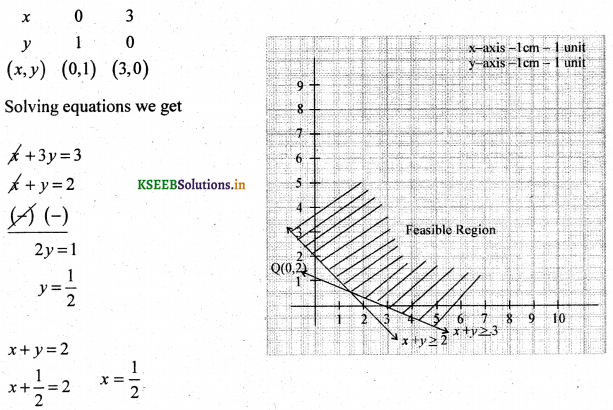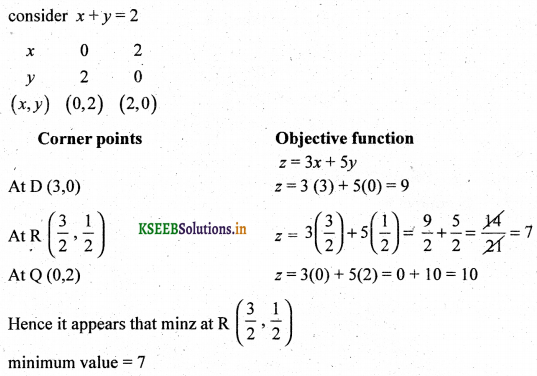Question 4.
Minimise Z = x – 7y + 190 subject to x + y≤ 8
x ≤ 5,y ≤ 5, x + y ≥ 4, x ≥ 0, y ≥ 0
Consider Inequalities as equalities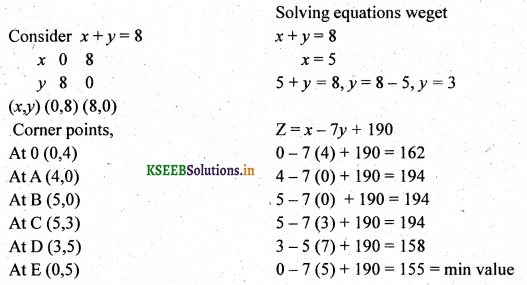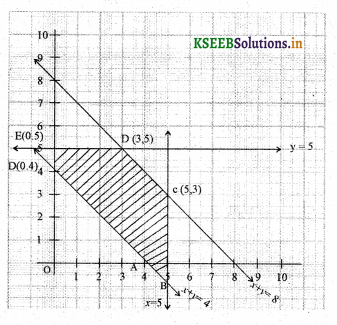Hence it appeases that Z min is at (0,5) and the minimum value is 155.

Question 5.
Kellogg is a new cereal formed from a mixture of bran & rice that contains at least 88 gram of protein and atleast 36 milligram of iron per kg knowing that bran contains 80 gm of protein & 40 milligram of iron per kg & that rice contains 100 gm of protein & 30 mg of iron per kg, find the minimum cost of producing this new cereal if bran cost ₹ 5 per kg & rice cost ₹ 4 per kg.
The formulated LPP is given by
Minimize z = 5x + 4y subject to the constraints
80x + 100y ≥ 88
40x + 30y ≥36
x ≥ 0,y ≥ 080x + 100y = 88
Put x = 0, then y = 1.1
Put y = 0 then x = 0.88
∴ The points are (0,1.1) (0.88,0)

40x + 30y = 36
Put x = 0, y= 0.9
Put y = 0 , x = 1.2
The points are (0,0.9) (1.2,0)

Solving equations
80x+ 100y = 88
40x + 30y = 36
we get x = 0.6 & y = 0.4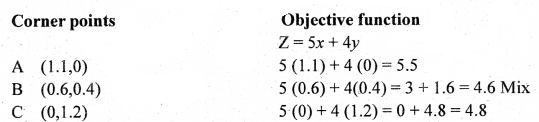∴ The optimal (min cost) solution is 4.6 when x = 0.6 kg of bran & y = 0.4kg of rice are mixed.Question 6.
There are two types of fertilizers F1 & F2. F1 consists of 10% nitrogen and 6% phosphoric acid and F2 consists of 5% Nitrogen and 10% phosphoric acid. After testing the soil conditions, a farmer vaidhya finds that he needs atleast 14 kg of N2 and 14 kg of phosphoric acid for the crop of F1 costs ₹6 per kg & F2 costs ₹5 per kg, determine how much of each type of fertilizer should be used so that ratrient requirements are met at a minimum cost, solve graphically.
The formatted LPP is given byMinimize z = 6x + 5y subject to the constraints
0.1x + 0.05 y ≥ 14
0.06x + 0.1y ≥ 14
x ≥ 0,y ≥ 0

Consider 0.1 x + 0.05y = 14          0.06x + 0.1y = 14
Put x = 0, then y = 280, (0, 280)    Put x = 0, y = 140,(0, 140)
Puty = 0 then x = 140, (140, 0)       Put y = 0,x = 233.3, (233,0)
Plot the points (0,280), (140,0) and (0,140), (233,0) on the grap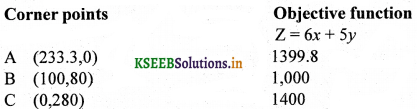∴ The optimal (minimum value) solution is 1000 when x = 100 and y = 80

Question 7.
A Television company owned by priyanka & B Bhavana operates Two assembly lines, line I & line II. Each of line is used to assemble the components of 3 types of Television colour standard and economy. The expected daily production on each line is as follows.The daily sunning costs from two lines average’ 6000 for line I and 4000 for line II, It is given that company must produce at least 24 colour, 16 std & 48 economy TV sets for which an order is pending. Formalte LPP & solve graphically determining the number of days the two lines should be run to meet the requirements.
The formulate LPP is given by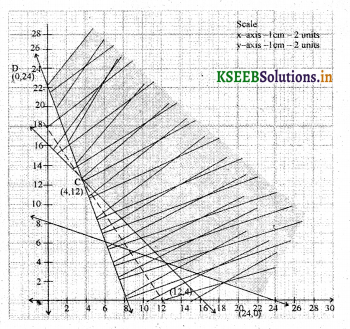Minimize Z = 6000x + 4000y subject to
3x + y ≥ 24
x+y = ≥ 16
2x + 6y ≥ 48
x ≥ 0,y ≥ 0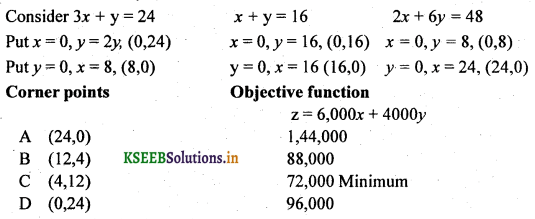∴ The optimal solution is z min = 72,000
When x = 4 and y = 12.Question 8.
Solve graphically
Maximize z = 30x + 40y
Subject to 3x + 6y ≤ 180
4x + 4y ≤ 200
x ≤ 0, y≤ 0
Consider the equalities
3x + 6y – 180             4x + 4y = 200
x – 0, y = 30, (0,30)     x = 0, y = 50, (0,50)
y = 0, x = 60 (60,0)    y = 0 ,x = 50, (50,0)
The feasible regions is bounded by OA BCQuestion 9.
Solve the following LP problem graphically minimize x = 5x + 7y subject to constraints
x + y ≤ 200
x + y ≤ 300
x ≥ 0, y ≥ 0
Consider the equalities
x + y = 200                                           x + y = 300
x = 0, y = 200, (0,200)                          x = 0, y = 300, (0,300)
y = 0, x = 200 (200,0)                           y = 0 , x = 300, (300,0)
Plot the points (0,200) (200,0) and (0,300) & (300,0) on the graph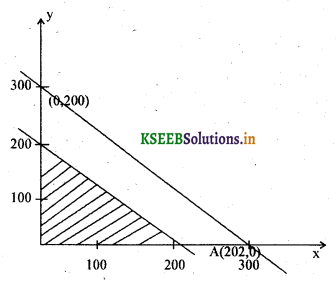Corner points Objective function
z = 5x + 7y
O (0,0)                           0
A (200,0)                       1000
B (0,200)                       1400
The optimal solution (minimum value) is 1000 at the points x = 0, y = 200Question 10.
Maximize Z = 200 x + 400y subject to
50x + 90y ≥ 3000
x+y ≤ 48
x,y ≤ 0
Consider the equalities
50x + 90y = 3000                            x + y = 48
x =10 ,$$y=\frac{100}{3},\left(0, \frac{100}{3}\right)$$   x = 0, y = 48, (0,48)
y = 0, x = 600(600,0)                       y = 0, x = 48, (48,0)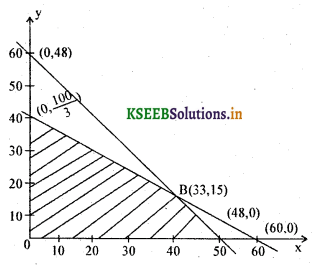Corner points Objective function

z = 200x + 400y
O(0,0)  z = 0
$$\mathrm{A}\left(0, \frac{100}{3}\right)$$ ≥ = 13333.3
B (33,15) ≥ = 12600
C (48,0)  ≥ = 9600
z is maximum at A
∴ the maximum profit is
obtained when x =0, y = 33.33